Courses

# Test: Dice- 2

## 10 Questions MCQ Test UPSC CSAT: Logical Reasoning (LR) & Data Interpretation (DI) | Test: Dice- 2

Description
This mock test of Test: Dice- 2 for UPSC helps you for every UPSC entrance exam. This contains 10 Multiple Choice Questions for UPSC Test: Dice- 2 (mcq) to study with solutions a complete question bank. The solved questions answers in this Test: Dice- 2 quiz give you a good mix of easy questions and tough questions. UPSC students definitely take this Test: Dice- 2 exercise for a better result in the exam. You can find other Test: Dice- 2 extra questions, long questions & short questions for UPSC on EduRev as well by searching above.
QUESTION: 1

### Directions to Solve Six dice with upper faces erased are as shows.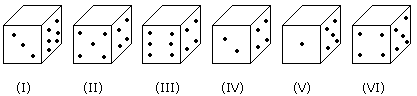The sum of the numbers of dots on the opposite face is 7. Question - If the odd numbered dice have even number of dots on their top faces, then what would be the total number of dots on the top faces of their dice?

Solution:

Odd numbered dice are : (I), (III) and (V)

No. of dots on the top faces of these dice are 2, 2 and 4 respectively.

Required total = 2 + 2 + 4 = 8

QUESTION: 2

### Directions to Solve Six dice with upper faces erased are as shows.The sum of the numbers of dots on the opposite face is 7. Question - If even numbered dice have even number of dots on their top faces, then what would be the total number of dots on the top faces of their dice?

Solution:

Even numbered dice are: (II), (IV) and (VI)

No. of dots on the top face of (II) dice = 6

No. of dots on the top face of (IV) dice = 6

and No. of dots on the top face of (VI) dice = 6

Therefore Required total = 6 + 6 + 6 = 18

QUESTION: 3

### Directions to Solve Six dice with upper faces erased are as shows.The sum of the numbers of dots on the opposite face is 7. Question - If dice (I), (II) and (III) have even number of dots on their bottom faces and the dice (IV), (V) and (VI) have odd number of dots on their top faces, then what would be the difference in the total number of top faces between there two sets?

Solution:

No. of faces on the top faces of the dice (I), (II) and (III) are 5, 1 and 5 respectively.

Therefore, Total of these numbers = 5 + 1 + 5 = 11

No. of dots on the top faces of the dice (IV), (V) and (VI) are 1, 3 and 1 respectively.

Therefore, Total of these numbers = 1 + 3 + 1 = 5

Required difference = 11 - 5 = 6

QUESTION: 4

Directions to Solve

Six dice with upper faces erased are as shows.The sum of the numbers of dots on the opposite face is 7.

Question -

If the even numbers of dice have odd number of dots on their top faces and odd numbered dice have even of dots on their bottom faces, then what would be the total number of dots on their top faces?

Solution:

No. of dots on the top faces of the dice (II), (IV) and (VI) are 1, 1 and 1 respectively.

No. of dots on the top faces of the dice (I), (III) and (V) are 5, 5 and 3 respectively.

Required total = 5 + 5 + 3 + 1 + 1 + 1 = 16

QUESTION: 5

Directions to Solve

Six dice with upper faces erased are as shows.The sum of the numbers of dots on the opposite face is 7.

Question -

If the dice (I), (II) and (III) have even number of dots on their bottom faces, then what would be the total number of dots on their top faces?

Solution:

No. of dots on the top faces of dice (I), (II) and (III) are 5, 1 and 5 respectively.

Required total = 5 + 1 + 5 = 11

QUESTION: 6

Directions to Solve

The figure given on the left hand side in each of the following questions is folded to form a box. Choose from the alternatives (1), (2), (3) and (4) the boxes that is similar to the box formed.

Question -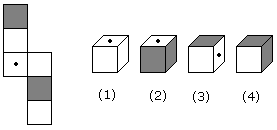Solution:

As, shown in figure, these are opposite of each other. Thus, option (2) is correct.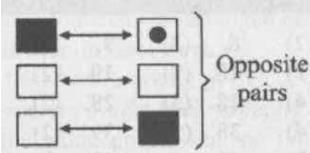QUESTION: 7

Directions to Solve

The figure given on the left hand side in each of the following questions is folded to form a box. Choose from the alternatives (1), (2), (3) and (4) the boxes that is similar to the box formed.

Question -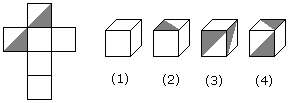Solution:
QUESTION: 8

Directions to Solve

The figure given on the left hand side in each of the following questions is folded to form a box. Choose from the alternatives (1), (2), (3) and (4) the boxes that is similar to the box formed.

Question -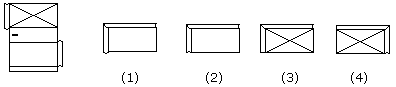Solution:

The pattern on fig. (X) and also the fact that the faces are rectangle, indicate that only fig. (1) can be obtained by folding fig. (X).

QUESTION: 9

Directions to Solve

The figure given on the left hand side in each of the following questions is folded to form a box. Choose from the alternatives (1), (2), (3) and (4) the boxes that is similar to the box formed.

Question -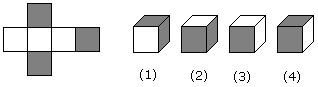Solution:
QUESTION: 10

Directions to Solve

The figure given on the left hand side in each of the following questions is folded to form a box. Choose from the alternatives (1), (2), (3) and (4) the boxes that is similar to the box formed.

Question -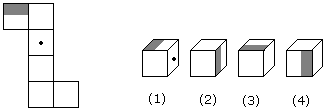Solution: